Get instant live expert help with Excel or Google Sheets“My Excelchat expert helped me in less than 20 minutes, saving me what would have been 5 hours of work!”

#### Post your problem and you’ll get expert help in seconds.

Your message must be at least 40 characters
Our professional experts are available now. Your privacy is guaranteed.

# How to Calculate a Weighted Average in an Excel Pivot Table

To calculate the weighted average of a data with a Pivot Table, we can add a column to our source data as an intermediate calculation. This is because Excel doesn’t provide a function in the Pivot Table that automatically calculates the weighted average. The steps below will walk through the process.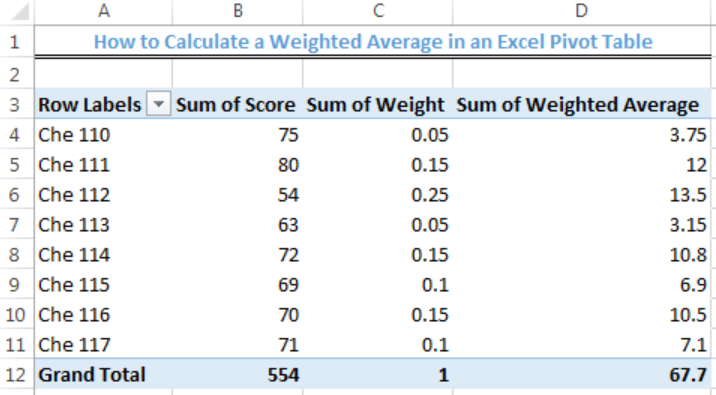Figure 1- How to Calculate a Weighted Average in an Excel Pivot Table

## Formula

`=B4*C4`

• Column B: These are the Scores in the data
• Column C: This is the percentage weight of each Course in the data

## Setting up the Data

We will calculate the weighted average score of the courses in the data below.

• Column A, B, and C will contain the Course, Score, and Weight respectively

We will use the formula above to calculate values for the weighted average column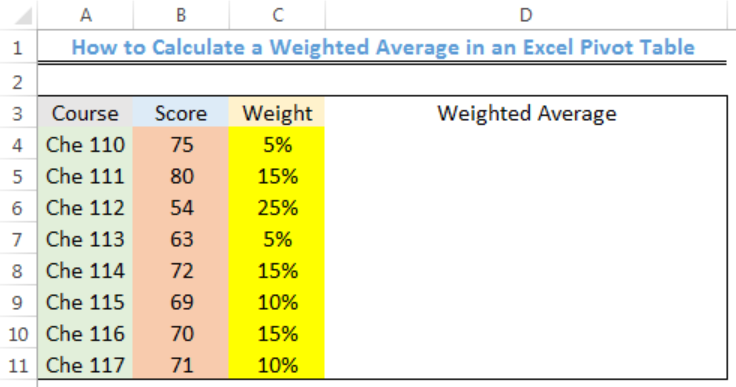Figure 2 – Setting up the Data

## Adding a Weighted Average Column to the Data

• We will click on Cell D4
• We will insert the formula below into Cell D4

`=B4*C4`

• We will press the enter key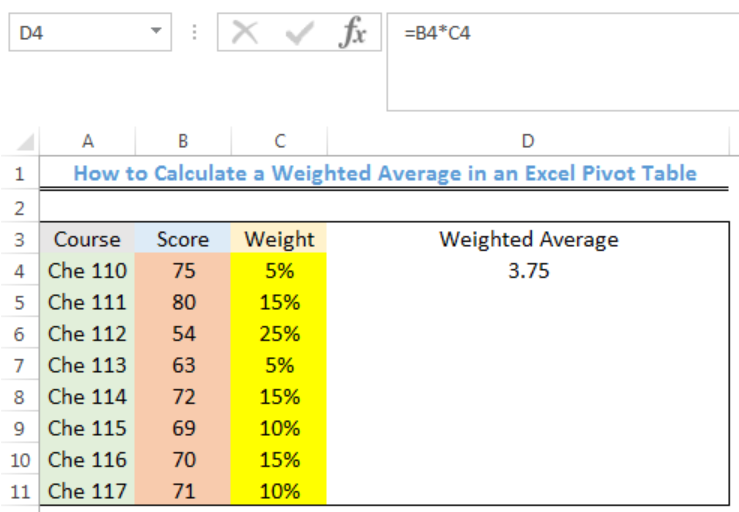Figure 3- Weighted Average Score for the Che 110

• We will click on Cell D4 again
• We will double click on the fill handle tool which is the small plus sign you see at the bottom right of Cell D4. We will select and drag down the fill handle to copy the formula to other cells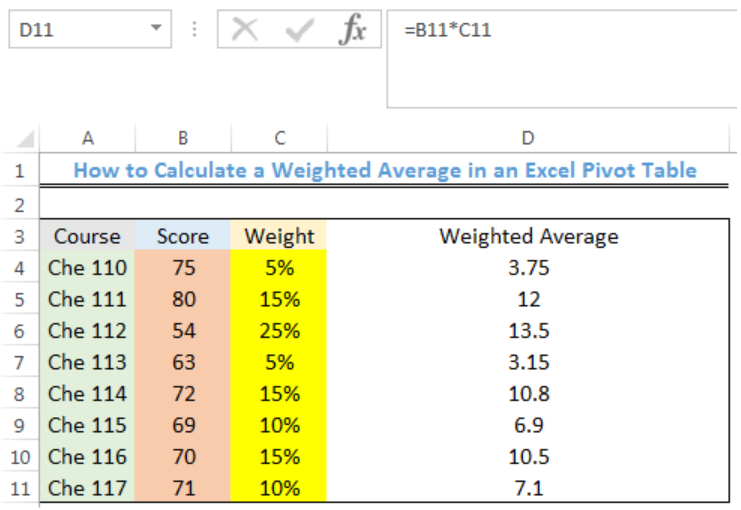Figure 4- Weighted Average Scores for the Courses

## Using the Pivot Table

• We will select the range (A3:D11) of the table
• We will click on the Insert tab and click on Pivot TableFigure 5- Clicking on Pivot Table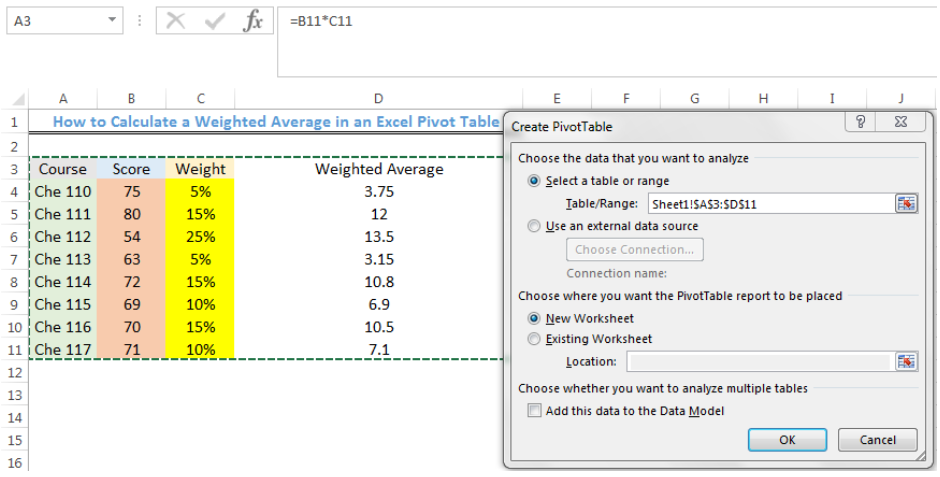Figure 6- Creating the Pivot Table

• We will press OK
• We will check the Pivot Table Fields to the right as shown in Figure 7Figure 7- Checking the Pivot Table Fields

• As we will see in figure 8, the Pivot Table has calculated the total weighted average as 67.7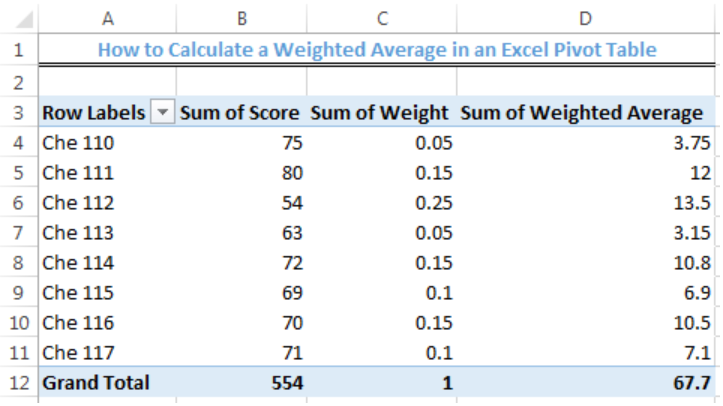Figure 8- Weighted Average Result from the Pivot Table

## Instant Connection to an Expert through our Excelchat Service

Most of the time, the problem you will need to solve will be more complex than a simple application of a formula or function. If you want to save hours of research and frustration, try our live Excelchat service! Our Excel Experts are available 24/7 to answer any Excel question you may have. We guarantee a connection within 30 seconds and a customized solution within 20 minutes.

Are you still looking for help with the Average function? View our comprehensive round-up of Average function tutorials here.